## Example Questions

1 2 3 5 Next →

### Example Question #41 : How To Find Data Representation In Physics

A scientist is exploring the nature of energy and work in two experimental systems.

Experiment 1

She first sets up a system with a ball on an inclined plane, and calculates the potential and kinetic energies of the ball at three positions. She places the ball at position 1, stops the ball after rolling to position 2, and then again allows it to roll to position 3. The measured kinetic and potential energies are shown in the table, along with measurements of the force of friction acting on the ball.

 Ball Position Potential Energy Kinetic Energy Force of Friction 1 150 kJ X kJ 0 N 2 75 kJ 75 kJ 0 N 3 X kJ 150 kJ 0 N

Experiment 2

The same scientist then sets up another experiment with the same ball, again at three positions. The ball is provided a slight push, and then allowed to roll down three levels without any additional external input of energy.

She uses the following formulae to calculate the energy levels of the ball:

Potential Energy = Mass of the Ball x Acceleration Due to Gravity x Height of the Ball

Kinetic Energy = (1/2) x Mass of the Ball x (Velocity of the Ball)2

She also measures the internal energy of the ball, a value that she defines as the amount of energy contained in the motion of the molecules that make up the matter of the ball.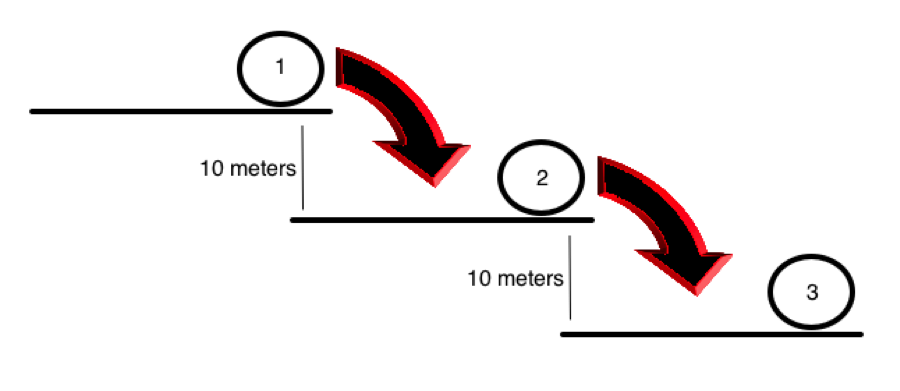Position Potential Energy Velocity Internal Energy 1 A 1 m/s 5 kJ 2 B 5.47 m/s 6 kJ 3 C 10.94 m/s 7 kJ

In Experiment 2, the scientist wishes to approximate the total loss of potential energy from position 1 (PE1) to position 3 (PE3). Which of the following is true?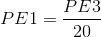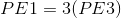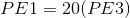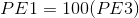Explanation:

The potential energy of the ball at position one is going to be 20 times higher than the potential energy of the ball at position 3 because the ball is 20 m higher. Potential energy, according to the provided formula, is dependent on this height for its magnitude.

### Example Question #1353 : Act Science

Experiment 1

A scientist develops the following setup, shown in Figure 1 below, to study the charges of radioactive particles. A radioactive sample is placed into a lead box that has an open column such that the particles can only exit from one direction. A detector is placed in front of the opening. A metric ruler measuring in centimeters (cm), is aligned on the detector such that zero is directly in front of the opening of the column, with positive values extending to the left and negative values extending to the right. On the left side of the experimental setup, there is a device that generates a magnetic field that attracts positively charged particles and repels negatively charged particles.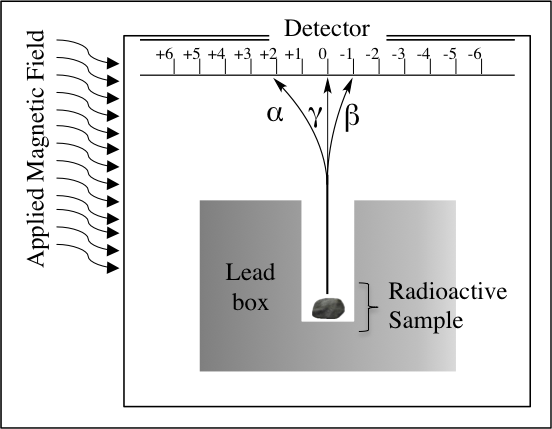Figure 1.

The device detects particles in three different places: alpha, α; beta, β; and gamma, γ; as labeled in Figure 1. The paths these particles take from the source of radioactivity are shown.

Experiment 2

A different scientist finds the following data, shown in Table 1, about the energies of the α, β, and γ particles by observing what kinds of materials through which the particles can pass. This scientist assumes that the ability of particles to pass through thicker and denser barriers is indicative of higher energy. Table 1 summarizes whether or not each type of particle was detected when each of the following barriers is placed between the radioactivity source and the detector. The paper and aluminum foil are both 1 millimeters thick, and the concrete wall is 1 meter thick.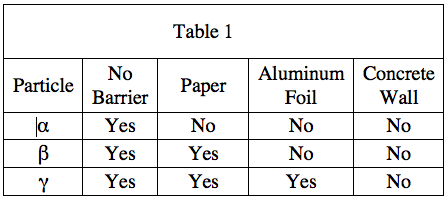Based on the trajectories of the particles in Experiment 1, what can be concluded about the relative charges of the particles?

The β particle must have a charge twice as large as the γ particle, as the applied magnetic field bent it twice as much.

The β particle must have a charge twice as large as the α particle, as the applied magnetic field bent it twice as much.

The α particle must have a charge twice as large as the γ particle, as the applied magnetic field bent it twice as much.

The α particle must have a charge twice as large as the β particle, as the applied magnetic field bent it twice as much.